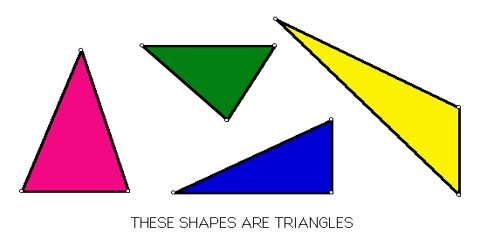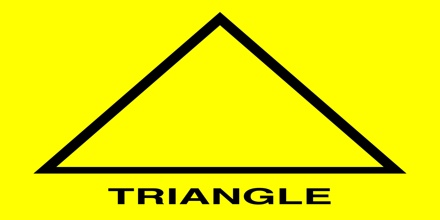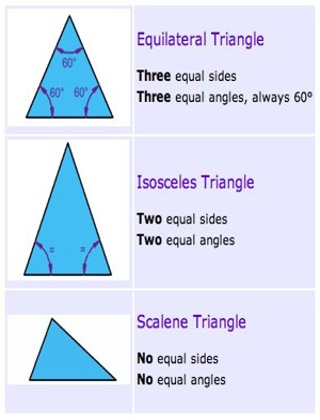Mathematic

# Triangle: Definition with TypesA triangle has three sides and three angles. The three angles always add to 180°

Every triangle has three sides and three angles, several of which may be similar. The sides of a triangle are given particular names in the case of a right triangle, with the side opposite the right angle being termed the hypotenuse and the other two sides being known as the legs. All triangles are convex and bicentric. That portion of the plane enclosed by the triangle is called the triangle interior, while the remainder is the exterior.Three main types (by angle) of triangle:

• Acute Triangle: All angles are less than 90°
• Right Triangle: Has a right angle (90°)
• Obtuse Triangle: Has an angle more than 90°Classifying triangles by their side lengths

• Equilateral triangles are triangles in which all three sides are the same length.
• Isosceles triangles are triangles in which two of the sides are the same length.
• Scalene triangles are triangles in which none of the sides are the same length.An angle cannot be obtuse and equilateral at the same time. An equilateral triangle cannot have an obtuse angle because all 3 angles in an equilateral triangle measure 60 degrees.